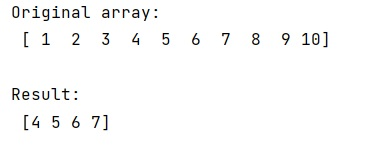# How to slice a numpy array along a dynamically specified axis?

Given a NumPy array, we have to slice it along a dynamically specified axis.
Submitted by Pranit Sharma, on February 15, 2023

NumPy is an abbreviated form of Numerical Python. It is used for different types of scientific operations in python. Numpy is a vast library in python which is used for almost every kind of scientific or mathematical operation. It is itself an array which is a collection of various methods and functions for processing the arrays.

## Slicing a numpy array along a dynamically specified axis

Suppose that we are given a numpy array and we need to slice this numpy array using a dynamically specified axis. By this, we mean that we will specify the axis, starting point, and ending point dynamically and then slice the array.

For this purpose, we will use numpy.arr.take() method which takes indices and axis as a parameter that can be assigned in order to slice the array dynamically.

Let us understand with the help of an example,

## Python code to slice a numpy array along a dynamically specified axis

```# Import numpy
import numpy as np

# Creating a numpy array
arr = np.array([1, 2, 3, 4, 5, 6,7,8,9,10])

# Display original array
print("Original array:\n",arr,"\n")

# Slicing this array using arr.take
res = arr.take(indices=[3,4,5,6], axis=0)

# Display result
print("Result:\n",res)
```

Output: